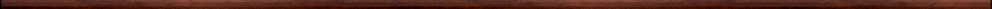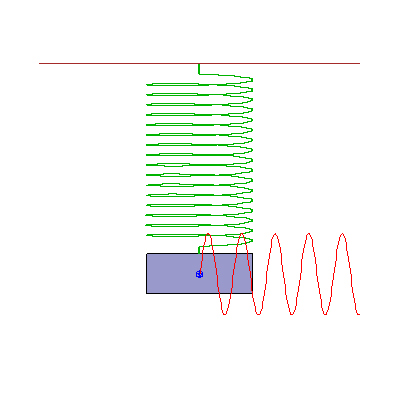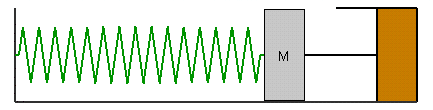Maple worksheets on second order differential equationsDifferential equations:

They are all compatible with Classic Worksheet Maple 10.Homogeneous 2nd order linear differential equations with constant coefficients - homog2DE.mws

• Introductory example.
• General method of solution of homogeneous 2nd order linear DE's with constant coefficients.
• The case where the auxiliary equation has equal roots.
• The case where the auxiliary equation has complex roots.
• Summary of standard forms for the solution.

Non-homogeneous 2nd order linear differential equations with constant coefficients - undetcoeff.mws

• The general form of a second order linear differential equation.
• General method of solution of non-homogeneous 2nd order linear DE's with constant coefficients.
• Introduction to the method of undetermined coefficients for determining a particular solution.
• A table of standard trial particular solutions.

Non-homogeneous 2nd order linear differential equations: variation of parameters - varparam.mws

• Determining a particular integral by variation of parameters: theory.
• Variation of parameters: summary of method.
• Variation of parameters: examples.

A procedure for solving 2nd order linear differential equations with constant coefficients - desolveCC.mws

• A procedure for solving 2nd order linear DE's with constant coefficients: desolveCC.
• Examples

Simple harmonic motion and damped oscillations - oscillations.mws

• Simple harmonic motion
• Damped motion
• Over-damped systems
• Critically damped systems
• Under-damped systems

Forced oscillations - forced.mws

• Applying an external force to a damped system.
• The transient and steady state parts of a solution.
• Resonance .. introductory example.
• Beats .. introductory example.
• Resonance as a limiting situation with respect to the natural frequency and the frequency of the forcing term.
• An under-damped system for which the frequency of the forcing term coincides with the natural frequency.

Resonance of an under-damped oscillatory system - resonance.mws

• Genuine resonance and resonant angular frequency .. an example.
• Genuine resonance and resonant angular frequency .. general case.
• The dependence of the resonant angular frequency on damping.
• Summary.

A procedure for solving 2nd order differential equations with a "missing variable" - missingvar.mws

• A procedure for solving 2nd order DE's by substituting for the derivative: desolveMV.
• Examples with the dependent variable missing.
• Examples with the independent variable missing.

Procedures for differential equations - DEsol.zipTop of page

Main index